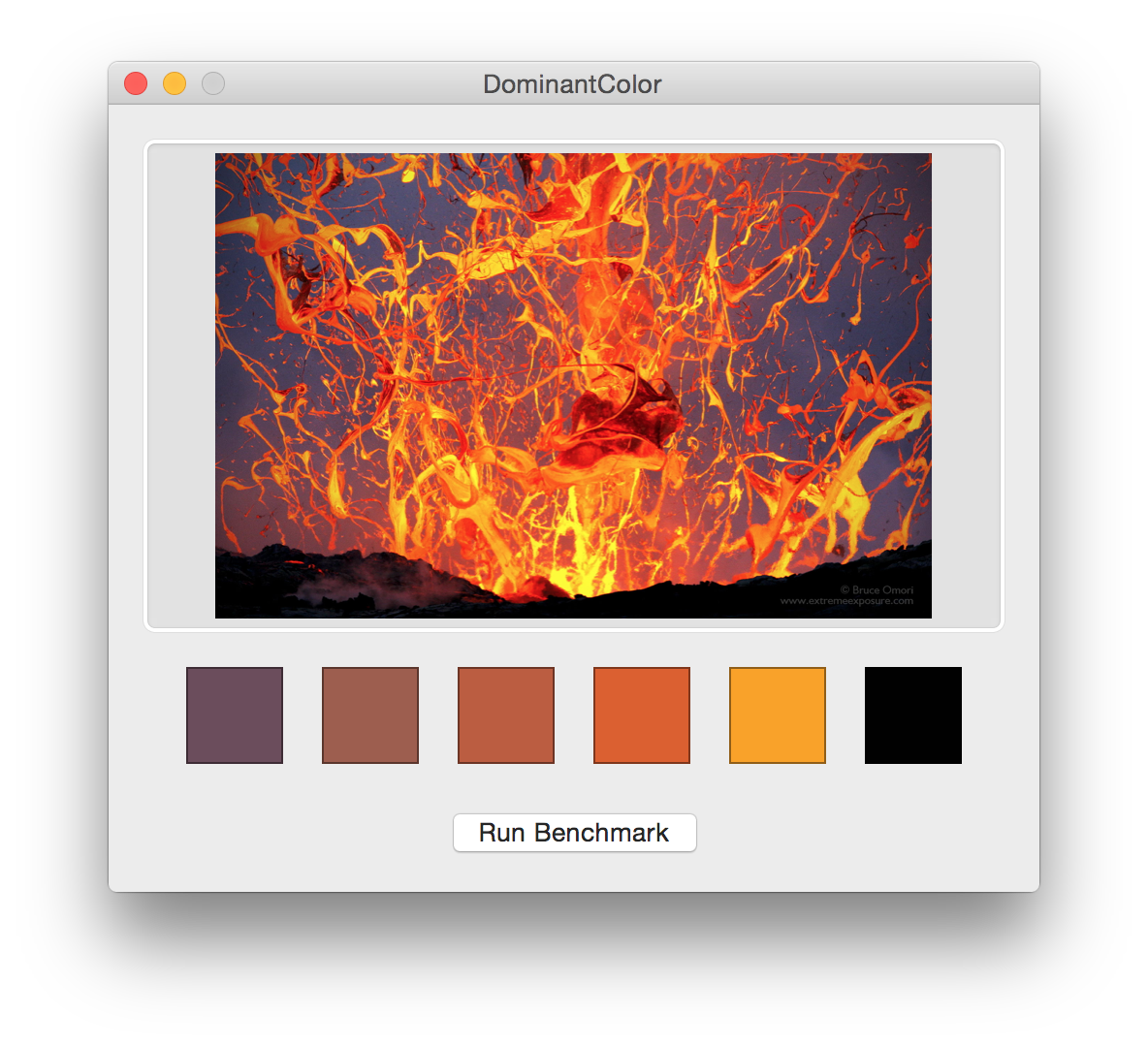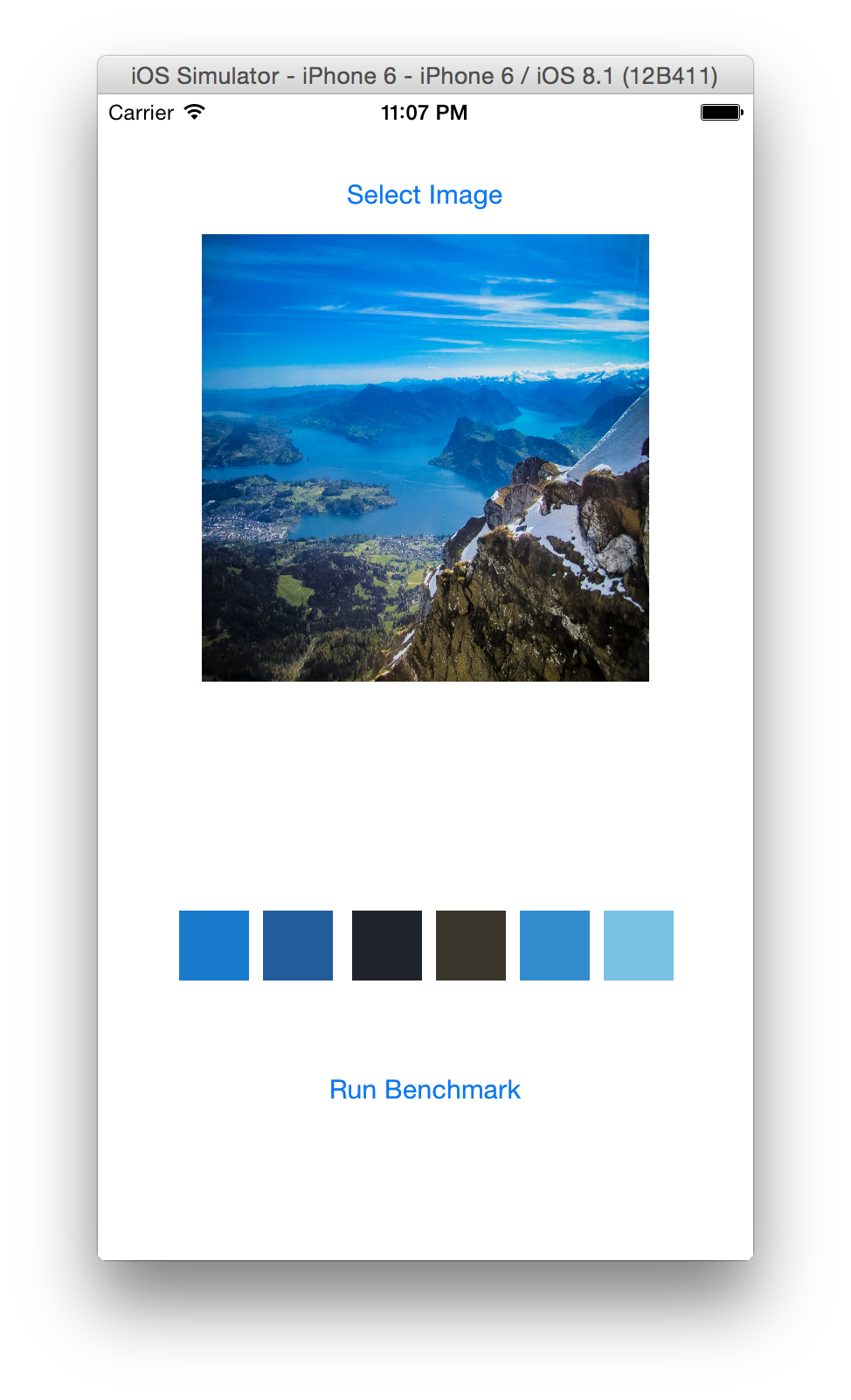Swiftpack.co -  indragiek/DominantColor as Swift Package
Swiftpack.co is a collection of thousands of indexed Swift packages. Search packages.
indragiek/DominantColor
Finding dominant colors of an image using k-means clustering
.package(url: "https://github.com/indragiek/DominantColor.git", from: "0.2.1")

## DominantColor

Finding the dominant colors of an image using the CIE LAB color space and the k-means clustering algorithm.

### Algorithm

#### Color Space

The RGB color space does not take human perception into account, so the CIELAB color space is used instead, which is designed to approximate human vision .

##### Conversion from RGB

The conversion from RGB values to LAB values requires first transforming the RGB values to an absolute color space like sRGB. On iOS, this conversion is not necessary because sRGB is the native device color space. On OS X, the conversion can be performed using `-[NSColorSpace sRGBColorSpace]`.

Once the colors have been converted to sRGB, they are first converted to linear sRGB values and then to CIE XYZ values. Finally, they are converted to the CIE LAB color space using a D65 standard illuminant.

#### Color Difference

A color difference algorithm is used to group similar colors. API consumers can choose between the CIE 76, CIE 94, and CIE 2000 algorithms for low, medium, and high color grouping accuracy, respectively. The default algorithm is CIE 94, as it provides results that are close to CIE 2000 with a negligible performance impact in comparison to CIE 76.

#### Clustering (k-means)

Pixels are grouped into clusters of dominant colors using a standard k-means clustering algorithm.

##### Choosing K

The k-value was originally chosen based on the rule of thumb `k = sqrt(n/2)` but this resulted in `k`-values that were too large to run in a reasonable amount of time for large values of `n`. Right now, I'm using a magic value of `16` because empirical testing showed that it yielded the best results for many different images but I'm still looking into a number of more data-driven alternate approaches.

##### Selecting Initial Centroids

The initial centroids are currently selected on a random basis. An alternative approach is to use the k-means++ algorithm, in which after the first centroid is selected randomly, the subsequent centroids are selected with probability proportional to the distance from the randomly selected centroid.

#### Downsampling

The k-means algorithm has a worst case runtime that is super-polynomial in the input size, so sampling large numbers of pixels is a problem. Images are automatically downsampled such that the total number of pixels is less than or equal to a specified maximum number of pixels to sample. The value I've been using is `1000`, which is a good balance between accurate results and runtime.

### Implementation

Everything is implemented in Swift except for the functions that convert between color spaces, which use GLKit and thus must be written in C (since Swift doesn't support C unions at this time).

### Apps

The project includes Mac and iOS apps that can be used to see the results of the algorithm and to run a simple benchmark.### GitHub

 link Stars: 848 Last commit: 1 week ago

Replace `GLKit` (deprecated) with `simd` and add Swift Package Manager support (#46), thanks @dagronf!